# MAFS.7.NS.1.1Archived StandardExport Print
Apply and extend previous understandings of addition and subtraction to add and subtract rational numbers; represent addition and subtraction on a horizontal or vertical number line diagram.
1. Describe situations in which opposite quantities combine to make 0. For example, a hydrogen atom has 0 charge because its two constituents are oppositely charged.
2. Understand p + q as the number located a distance |q| from p, in the positive or negative direction depending on whether q is positive or negative. Show that a number and its opposite have a sum of 0 (are additive inverses). Interpret sums of rational numbers by describing real-world contexts.
3. Understand subtraction of rational numbers as adding the additive inverse, p – q = p + (–q). Show that the distance between two rational numbers on the number line is the absolute value of their difference, and apply this principle in real-world contexts.
4. Apply properties of operations as strategies to add and subtract rational numbers.

### Remarks

Fluency Expectations or Examples of Culminating Standards

Adding, subtracting, multiplying, and dividing rational numbers is the culmination of numerical work with the four basic operations. The number system will continue to develop in grade 8, expanding to become the real numbers by the introduction of irrational numbers, and will develop further in high school, expanding to become the complex numbers with the introduction of imaginary numbers. Because there are no specific standards for rational number arithmetic in later grades and because so much other work in grade 7 depends on rational number arithmetic, fluency with rational number arithmetic should be the goal in grade 7.
General Information
Subject Area: Mathematics
Domain-Subdomain: The Number System
Cluster: Level 2: Basic Application of Skills & Concepts
Cluster: Apply and extend previous understandings of operations with fractions to add, subtract, multiply, and divide rational numbers. (Major Cluster) -

Clusters should not be sorted from Major to Supporting and then taught in that order. To do so would strip the coherence of the mathematical ideas and miss the opportunity to enhance the major work of the grade with the supporting clusters.

Date of Last Rating: 02/14
Status: State Board Approved - Archived
Assessed: Yes
Test Item Specifications

• Assessment Limits :

N/A

• Calculator :

Neutral

• Context :

Allowable

Sample Test Items (7)
• Test Item #: Sample Item 1
• Question:

A number line is shown.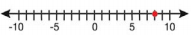Use the Add Point tool to plot a point that is 14.5 units from 8 on the given number line.

• Difficulty: N/A
• Type: GRID: Graphic Response Item Display

• Test Item #: Sample Item 2
• Question:

An expression is shown.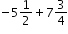What is the value of the expression?

• Difficulty: N/A
• Type: EE: Equation Editor

• Test Item #: Sample Item 3
• Question:

A number line is shown.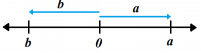Jack knows that a + b = 0.

Which statement is true?

• Difficulty: N/A
• Type: MC: Multiple Choice

• Test Item #: Sample Item 4
• Question:

An expression is shown.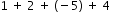Kendrick is using number lines to find the value of the expression. His first two steps are shown.

A. Use the Add Arrow tool to show the last two steps.

B. Select the value of the expression.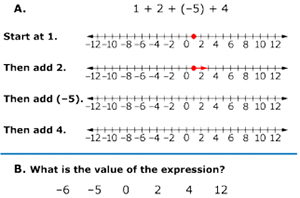• Difficulty: N/A
• Type: GRID: Graphic Response Item Display

• Test Item #: Sample Item 5
• Question:

An expression is shown.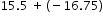What is the value of the expression?

• Difficulty: N/A
• Type: EE: Equation Editor

• Test Item #: Sample Item 6
• Question:

The sum of a and b is c. The number line shows a and b.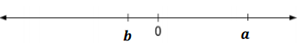Which statements about c are true?

• Difficulty: N/A
• Type: MS: Multiselect

## Related Courses

This benchmark is part of these courses.
1205020: M/J Accelerated Mathematics Grade 6 (Specifically in versions: 2014 - 2015, 2015 - 2020, 2020 - 2022, 2022 and beyond (current))
1205040: M/J Grade 7 Mathematics (Specifically in versions: 2014 - 2015, 2015 - 2022, 2022 and beyond (current))
1204000: M/J Foundational Skills in Mathematics 6-8 (Specifically in versions: 2014 - 2015, 2015 - 2022, 2022 and beyond (current))
1200410: Mathematics for College Success (Specifically in versions: 2014 - 2015, 2015 - 2022 (course terminated))
1200700: Mathematics for College Algebra (Specifically in versions: 2014 - 2015, 2015 - 2022 (course terminated))
7812020: Access M/J Grade 7 Mathematics (Specifically in versions: 2014 - 2015, 2015 - 2018, 2018 - 2019, 2019 - 2022, 2022 and beyond (current))
7912115: Fundamental Explorations in Mathematics 2 (Specifically in versions: 2013 - 2015, 2015 - 2017 (course terminated))

## Related Access Points

Alternate version of this benchmark for students with significant cognitive disabilities.

## Related Resources

Vetted resources educators can use to teach the concepts and skills in this benchmark.

## Educational Games

Pinata Fever: A Visual Integer Sums Number Line Game:

Practice adding and subtracting positive and negative numbers to intercept the descending piñatas and then whack them for candy. Come on, keep the party going!

Type: Educational Game

Integers Jeopardy Game:

This interactive game has 4 categories: adding integers, subtracting integers, multiplying integers, and dividing integers. Students can play individually or in teams.

Type: Educational Game

## Formative Assessments

Using Positive and Negative Numbers in Context:

This lesson unit is intended to help you assess how well students are able to understand and use directed numbers in context. It is intended to help identify and aid students who have difficulties in ordering, comparing, adding, and subtracting positive and negative integers. Particular attention is paid to the use of negative numbers on number lines to explore the structures:

• starting temperature + change in temperature = final temperature
• final temperature – change in temperature = starting temperature
• final temperature – starting temperature = change in temperature

Type: Formative Assessment

Type: Formative Assessment

Rational Water Management:

Students are asked to combine rational numbers, including fractions and decimals, and use the properties of operations to simplify calculations.

Type: Formative Assessment

Finding the Difference:

Students are asked to find the difference between two integers using a number line.

Type: Formative Assessment

Students are asked to rewrite a subtraction problem as an equivalent addition problem and explain the equivalence using a number line.

Type: Formative Assessment

Students are asked to describe an example of additive inverse and demonstrate the concept on a number line.

Type: Formative Assessment

## Lesson Plans

Based on a student-focused scenario encouraging healthier lifestyles, students will perform a close and careful reading of an article encouraging active and healthy lifestyles. During the lesson, students will analyze data from Consumer Reports comparing and contrasting treadmills and elliptical exercisers. Using information gathered, students will compile data and persuade administrators to buy equipment that will align with the provided budget and fit in the given space.

Type: Lesson Plan

Using Positive and Negative Numbers in Context:

This lesson unit is intended to help you assess how well students are able to understand and use directed numbers in context. It is intended to help identify and aid students who have difficulties in ordering, comparing, adding, and subtracting positive and negative integers. Particular attention is paid to the use of negative numbers on number lines to explore the following structures:

• starting temperature + change in temperature = final temperature
• final temperature – change in temperature = starting temperature
• final temperature – starting temperature = change in temperature

Type: Lesson Plan

Discovering How to Subtract Rational Numbers Using the Additive Inverse:

In this lesson, students will develop an understanding that opposite quantities combine to make zero and will learn how to subtract rational numbers using a horizontal number line and the additive inverse

Type: Lesson Plan

In this lesson, students will use T-charts to add and subtract positive and negative numbers.

Type: Lesson Plan

Discovering our Addition of Integer Rules:

In this lesson, students will develop the rules for adding integers by using the absolute value of integers and number lines.

Type: Lesson Plan

## Original Student Tutorial

Learn how to explain the meaning of additive inverse, identify the additive inverse of a given rational number, and justify your answer on a number line in this original tutorial.

Type: Original Student Tutorial

Distances on the Number Line 2:

The purpose of this task is meant to reinforce students' understanding of rational numbers as points on the number line and to provide them with a visual way of understanding that the sum of a number and its additive inverse (usually called its "opposite") is zero.

Comparing Freezing Points:

In this task, students answer a question about the difference between two temperatures that are negative numbers.

Operations on the Number Line:

The purpose of this task is to help solidify students' understanding of signed numbers as points on a number line and to understand the geometric interpretation of adding and subtracting signed numbers. There is a subtle distinction in the academic standards between a fraction and a rational number. Fractions are always positive, and when thinking of the symbol ab as a fraction, it is possible to interpret it as a equal-sized pieces where b pieces make one whole.

## Teaching Idea

This video offers a quick and easy way for math students to add numbers with positive and negative signs by modelling with colored stickers. The teacher used red stickers for negative numbers, orange as a neutral, and green for positives in order to give the students a visual learning tool to help tackle the world of integer addition.

Type: Teaching Idea

## Tutorials

Adding and Subtracting Numbers in Different Formats:

In this example, we will work with three numbers in different formats: a percent, a decimal, and a mixed number.

Type: Tutorial

Simplifying Expressions with Rational Numbers:

In this tutorial, you will simplify expressions involving positive and negative fractions.

Type: Tutorial

Rational Number Word Problem with Decimals:

This video demonstrates adding and subtracting decimals in the context of an overdrawn checking account.

Type: Tutorial

Substitution with negative numbers:

Practice substituting positive and negative values for variables.

Type: Tutorial

Finding the absolute value as distance between numbers:

In this video, we will find the absolute value as distance between rational numbers.

Type: Tutorial

Creating equivalent expressions with positive and negative numbers:

This video will demonstrate how to create quivalent expressions with positive and negative numbers.

Type: Tutorial

Even More Negative Number Practice:

This video uses the number line to find unknown values in subtraction statements with negative numbers.

Type: Tutorial

Adding Negative Numbers on Number Line Examples:

This video asks you to select the model that matches the given expression.

Type: Tutorial

Negative Number Word Problem:

Use a number line to solve a word problem that includes a negative number.

Type: Tutorial

Finding Initial Temperature from Temperature Changes:

In this video, we figure out the temperature in Fairbanks, Alaska by adding and subtracting integers.

Type: Tutorial

This video demonstrates how to add and subtract negative fractions with unlike denominators.

Type: Tutorial

This video demonstrates use of a number line and absolute value to add negative numbers.

Type: Tutorial

This video demonstrates use of a number line to add numbers with positive and negative signs.

Type: Tutorial

Subtracting a Negative = Adding a Positive:

Find out why subtracting a negative number is the same as adding the absolute value of that number.

Type: Tutorial

Negative Number Practice:

This video demonstrates adding and subtracting integers using a number line.

Type: Tutorial

Pre-Algebra - Commutative & Associative Properties of Addition:

A look behind the fundamental properties of the most basic arithmetic operation, addition

Type: Tutorial

Students will be able to see examples of addition of integers while watching a short video, and practice adding integers using an online quiz.

Type: Tutorial

## Video/Audio/Animations

Interpreting Integer Expressions on the Number Line:

This video contains three examples of interpreting integer expressions in the context of a number line.

Type: Video/Audio/Animation

Interpreting Negative Number Statements:

Explore negative numbers to represent real world situations in this tutorial.

Type: Video/Audio/Animation

## Virtual Manipulative

Math Match - Concepts Review Game:

This interactive game allows students to review math concepts, including shapes, shape names, addition, multiplication, negative numbers, and equivalent expressions.

Type: Virtual Manipulative

## STEM Lessons - Model Eliciting Activity

Based on a student-focused scenario encouraging healthier lifestyles, students will perform a close and careful reading of an article encouraging active and healthy lifestyles. During the lesson, students will analyze data from Consumer Reports comparing and contrasting treadmills and elliptical exercisers. Using information gathered, students will compile data and persuade administrators to buy equipment that will align with the provided budget and fit in the given space.

## MFAS Formative Assessments

Students are asked to describe an example of additive inverse and demonstrate the concept on a number line.

Finding the Difference:

Students are asked to find the difference between two integers using a number line.

Students are asked to rewrite a subtraction problem as an equivalent addition problem and explain the equivalence using a number line.

Rational Water Management:

Students are asked to combine rational numbers, including fractions and decimals, and use the properties of operations to simplify calculations.

## Original Student Tutorials Mathematics - Grades 6-8

Learn how to explain the meaning of additive inverse, identify the additive inverse of a given rational number, and justify your answer on a number line in this original tutorial.

## Student Resources

Vetted resources students can use to learn the concepts and skills in this benchmark.

## Original Student Tutorial

Learn how to explain the meaning of additive inverse, identify the additive inverse of a given rational number, and justify your answer on a number line in this original tutorial.

Type: Original Student Tutorial

## Educational Game

Integers Jeopardy Game:

This interactive game has 4 categories: adding integers, subtracting integers, multiplying integers, and dividing integers. Students can play individually or in teams.

Type: Educational Game

Distances on the Number Line 2:

The purpose of this task is meant to reinforce students' understanding of rational numbers as points on the number line and to provide them with a visual way of understanding that the sum of a number and its additive inverse (usually called its "opposite") is zero.

Comparing Freezing Points:

In this task, students answer a question about the difference between two temperatures that are negative numbers.

Operations on the Number Line:

The purpose of this task is to help solidify students' understanding of signed numbers as points on a number line and to understand the geometric interpretation of adding and subtracting signed numbers. There is a subtle distinction in the academic standards between a fraction and a rational number. Fractions are always positive, and when thinking of the symbol ab as a fraction, it is possible to interpret it as a equal-sized pieces where b pieces make one whole.

## Tutorials

Adding and Subtracting Numbers in Different Formats:

In this example, we will work with three numbers in different formats: a percent, a decimal, and a mixed number.

Type: Tutorial

Simplifying Expressions with Rational Numbers:

In this tutorial, you will simplify expressions involving positive and negative fractions.

Type: Tutorial

Rational Number Word Problem with Decimals:

This video demonstrates adding and subtracting decimals in the context of an overdrawn checking account.

Type: Tutorial

Substitution with negative numbers:

Practice substituting positive and negative values for variables.

Type: Tutorial

Finding the absolute value as distance between numbers:

In this video, we will find the absolute value as distance between rational numbers.

Type: Tutorial

Even More Negative Number Practice:

This video uses the number line to find unknown values in subtraction statements with negative numbers.

Type: Tutorial

Adding Negative Numbers on Number Line Examples:

This video asks you to select the model that matches the given expression.

Type: Tutorial

Negative Number Word Problem:

Use a number line to solve a word problem that includes a negative number.

Type: Tutorial

Finding Initial Temperature from Temperature Changes:

In this video, we figure out the temperature in Fairbanks, Alaska by adding and subtracting integers.

Type: Tutorial

This video demonstrates how to add and subtract negative fractions with unlike denominators.

Type: Tutorial

This video demonstrates use of a number line and absolute value to add negative numbers.

Type: Tutorial

This video demonstrates use of a number line to add numbers with positive and negative signs.

Type: Tutorial

Subtracting a Negative = Adding a Positive:

Find out why subtracting a negative number is the same as adding the absolute value of that number.

Type: Tutorial

Negative Number Practice:

This video demonstrates adding and subtracting integers using a number line.

Type: Tutorial

Pre-Algebra - Commutative & Associative Properties of Addition:

A look behind the fundamental properties of the most basic arithmetic operation, addition

Type: Tutorial

Students will be able to see examples of addition of integers while watching a short video, and practice adding integers using an online quiz.

Type: Tutorial

## Parent Resources

Vetted resources caregivers can use to help students learn the concepts and skills in this benchmark.

Distances on the Number Line 2:

The purpose of this task is meant to reinforce students' understanding of rational numbers as points on the number line and to provide them with a visual way of understanding that the sum of a number and its additive inverse (usually called its "opposite") is zero.

Comparing Freezing Points:

In this task, students answer a question about the difference between two temperatures that are negative numbers.

Operations on the Number Line:

The purpose of this task is to help solidify students' understanding of signed numbers as points on a number line and to understand the geometric interpretation of adding and subtracting signed numbers. There is a subtle distinction in the academic standards between a fraction and a rational number. Fractions are always positive, and when thinking of the symbol ab as a fraction, it is possible to interpret it as a equal-sized pieces where b pieces make one whole.